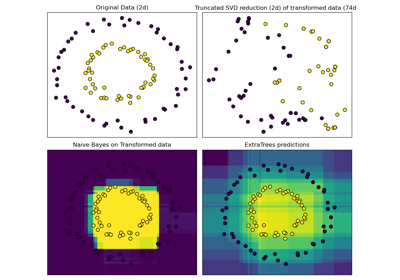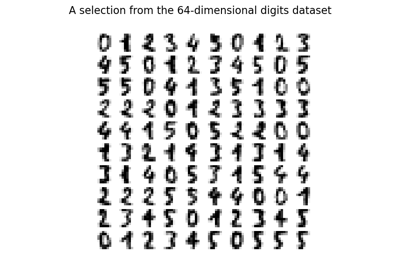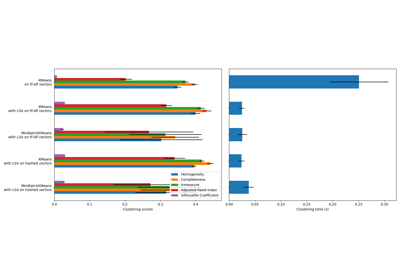# sklearn.decomposition.TruncatedSVD¶

class sklearn.decomposition.TruncatedSVD(n_components=2, *, algorithm='randomized', n_iter=5, random_state=None, tol=0.0)[source]

Dimensionality reduction using truncated SVD (aka LSA).

This transformer performs linear dimensionality reduction by means of truncated singular value decomposition (SVD). Contrary to PCA, this estimator does not center the data before computing the singular value decomposition. This means it can work with sparse matrices efficiently.

In particular, truncated SVD works on term count/tf-idf matrices as returned by the vectorizers in sklearn.feature_extraction.text. In that context, it is known as latent semantic analysis (LSA).

This estimator supports two algorithms: a fast randomized SVD solver, and a “naive” algorithm that uses ARPACK as an eigensolver on X * X.T or X.T * X, whichever is more efficient.

Read more in the User Guide.

Parameters
n_componentsint, default=2

Desired dimensionality of output data. Must be strictly less than the number of features. The default value is useful for visualisation. For LSA, a value of 100 is recommended.

algorithm{‘arpack’, ‘randomized’}, default=’randomized’

SVD solver to use. Either “arpack” for the ARPACK wrapper in SciPy (scipy.sparse.linalg.svds), or “randomized” for the randomized algorithm due to Halko (2009).

n_iterint, default=5

Number of iterations for randomized SVD solver. Not used by ARPACK. The default is larger than the default in randomized_svd to handle sparse matrices that may have large slowly decaying spectrum.

random_stateint, RandomState instance or None, default=None

Used during randomized svd. Pass an int for reproducible results across multiple function calls. See Glossary.

tolfloat, default=0.

Tolerance for ARPACK. 0 means machine precision. Ignored by randomized SVD solver.

Attributes
components_ndarray of shape (n_components, n_features)
explained_variance_ndarray of shape (n_components,)

The variance of the training samples transformed by a projection to each component.

explained_variance_ratio_ndarray of shape (n_components,)

Percentage of variance explained by each of the selected components.

singular_values_ndarray od shape (n_components,)

The singular values corresponding to each of the selected components. The singular values are equal to the 2-norms of the n_components variables in the lower-dimensional space.

Notes

SVD suffers from a problem called “sign indeterminacy”, which means the sign of the components_ and the output from transform depend on the algorithm and random state. To work around this, fit instances of this class to data once, then keep the instance around to do transformations.

References

Finding structure with randomness: Stochastic algorithms for constructing approximate matrix decompositions Halko, et al., 2009 (arXiv:909) https://arxiv.org/pdf/0909.4061.pdf

Examples

>>> from sklearn.decomposition import TruncatedSVD
>>> from scipy.sparse import random as sparse_random
>>> X = sparse_random(100, 100, density=0.01, format='csr',
...                   random_state=42)
>>> svd = TruncatedSVD(n_components=5, n_iter=7, random_state=42)
>>> svd.fit(X)
TruncatedSVD(n_components=5, n_iter=7, random_state=42)
>>> print(svd.explained_variance_ratio_)
[0.0646... 0.0633... 0.0639... 0.0535... 0.0406...]
>>> print(svd.explained_variance_ratio_.sum())
0.286...
>>> print(svd.singular_values_)
[1.553... 1.512...  1.510... 1.370... 1.199...]


Methods

 fit(X[, y]) Fit model on training data X. fit_transform(X[, y]) Fit model to X and perform dimensionality reduction on X. get_params([deep]) Get parameters for this estimator. Transform X back to its original space. set_params(**params) Set the parameters of this estimator. Perform dimensionality reduction on X.
fit(X, y=None)[source]

Fit model on training data X.

Parameters
X{array-like, sparse matrix} of shape (n_samples, n_features)

Training data.

yIgnored
Returns
selfobject

Returns the transformer object.

fit_transform(X, y=None)[source]

Fit model to X and perform dimensionality reduction on X.

Parameters
X{array-like, sparse matrix} of shape (n_samples, n_features)

Training data.

yIgnored
Returns
X_newndarray of shape (n_samples, n_components)

Reduced version of X. This will always be a dense array.

get_params(deep=True)[source]

Get parameters for this estimator.

Parameters
deepbool, default=True

If True, will return the parameters for this estimator and contained subobjects that are estimators.

Returns
paramsdict

Parameter names mapped to their values.

inverse_transform(X)[source]

Transform X back to its original space.

Returns an array X_original whose transform would be X.

Parameters
Xarray-like of shape (n_samples, n_components)

New data.

Returns
X_originalndarray of shape (n_samples, n_features)

Note that this is always a dense array.

set_params(**params)[source]

Set the parameters of this estimator.

The method works on simple estimators as well as on nested objects (such as Pipeline). The latter have parameters of the form <component>__<parameter> so that it’s possible to update each component of a nested object.

Parameters
**paramsdict

Estimator parameters.

Returns
selfestimator instance

Estimator instance.

transform(X)[source]

Perform dimensionality reduction on X.

Parameters
X{array-like, sparse matrix} of shape (n_samples, n_features)

New data.

Returns
X_newndarray of shape (n_samples, n_components)

Reduced version of X. This will always be a dense array.

## Examples using sklearn.decomposition.TruncatedSVD¶